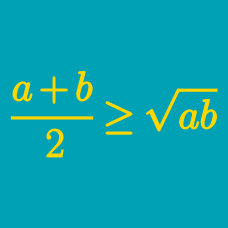Algebra

# Power Mean Inequalities: Level 4 Challenges

Find the minimum value of $x^6 + y^6 -54xy$, where $x$ and $y$ are real numbers.

Let $x, y, z$ be real numbers such that $x^{2}+y^{2}+z^{2}=1$. Let the maximum possible value of $\sqrt {6} xy+4yz$ be $A$.Find $A^{2}$.

###### This problem is inspired by Joel Tan.

Given that $x$, $y$, and $z$ are positive real numbers satisfying $xyz(x+y+z)=1$, minimize $(x+y)(y+z)(z+x)$.

$a, b$ and $c$ are positive real numbers greater than or equal to 1 satisfying

\begin{aligned} abc & =100,\\ a^{\lg a} b^{\lg b} c^{\lg c} & \geq 10000. \\ \end{aligned}

What is the value of $a + b + c$?

Details and assumptions:

• $\lg$ refers to log base 10.

For positive real numbers $a, b, c$, find the minimum integer value possible of the following equation:

$6a^{3} + 9b^{3} + 32c^{3} + \frac{1}{4abc}$